Ex 3.2

Chapter 3 Class 10 Pair of Linear Equations in Two Variables
Serial order wise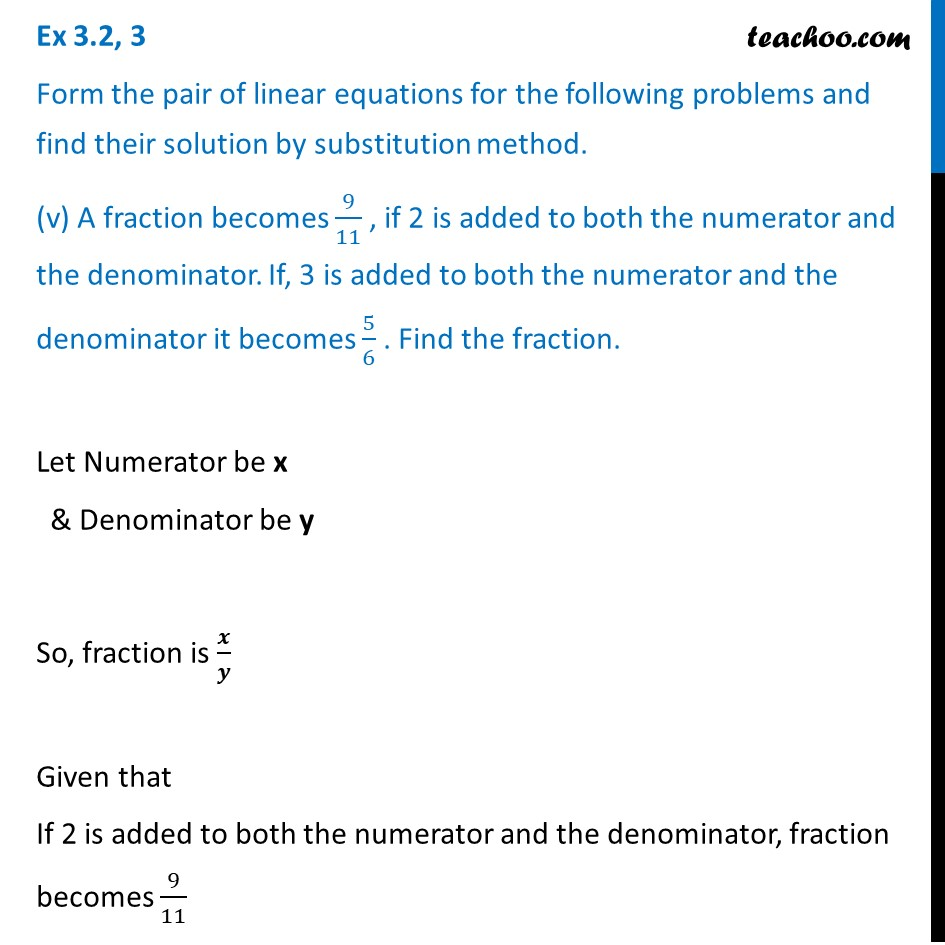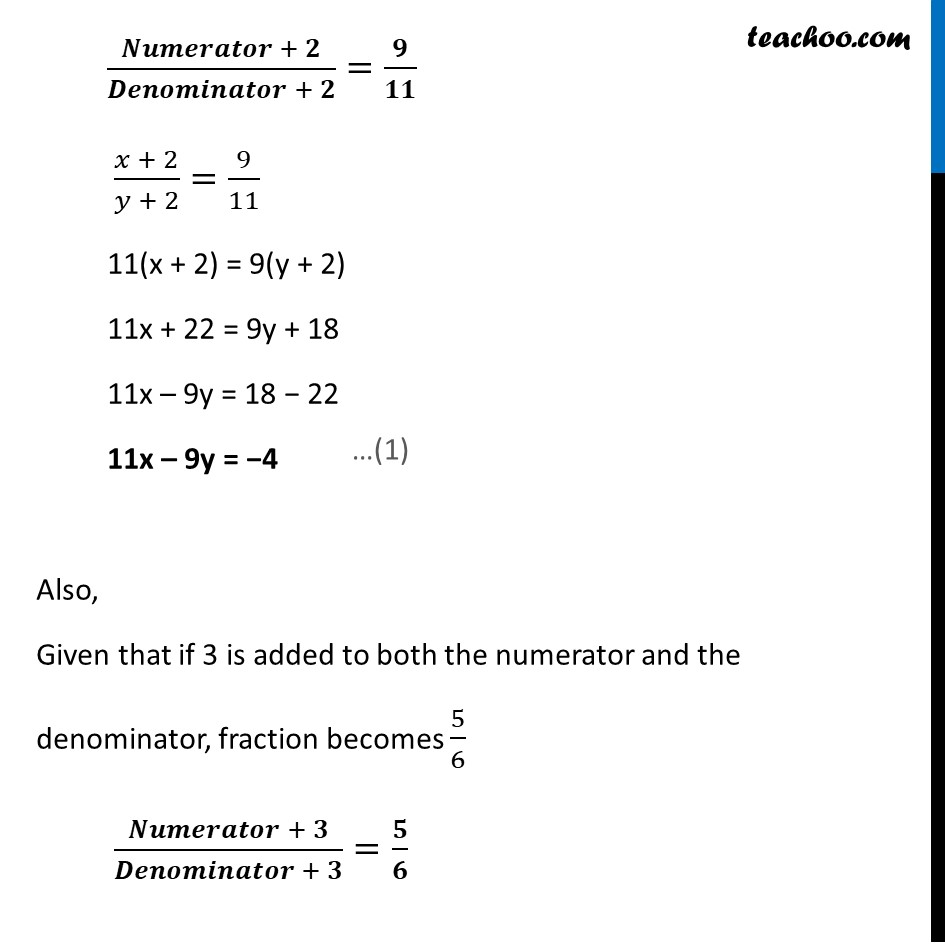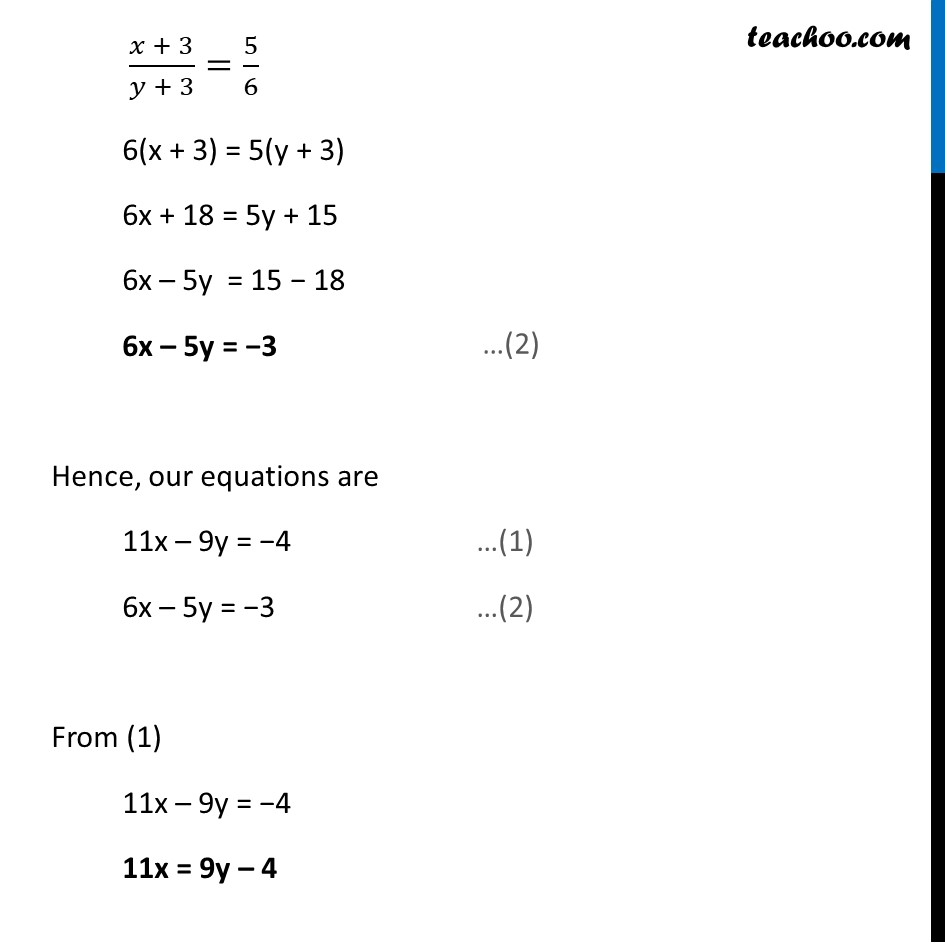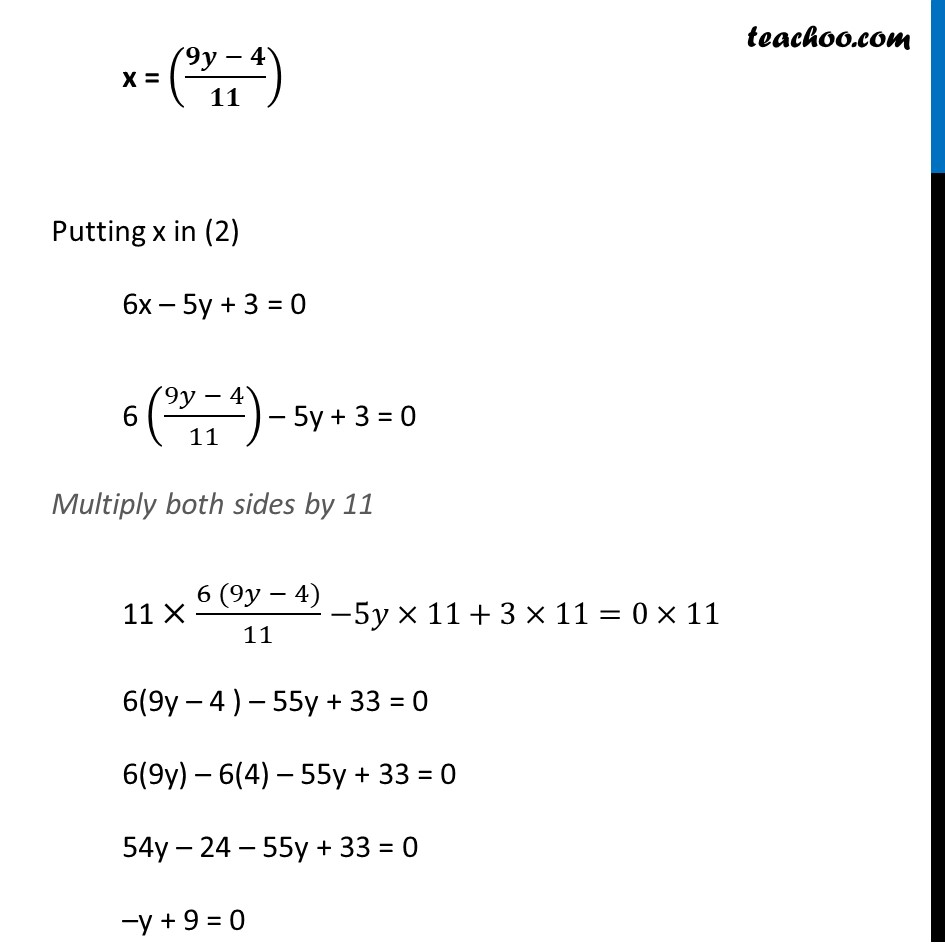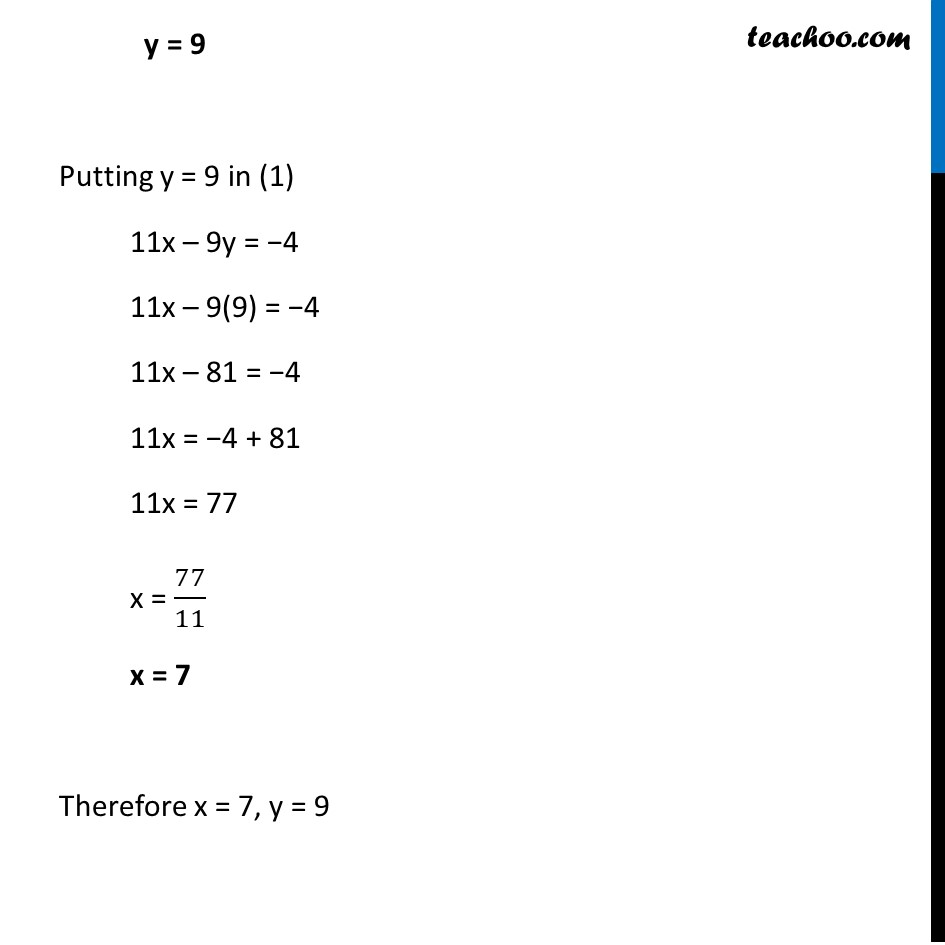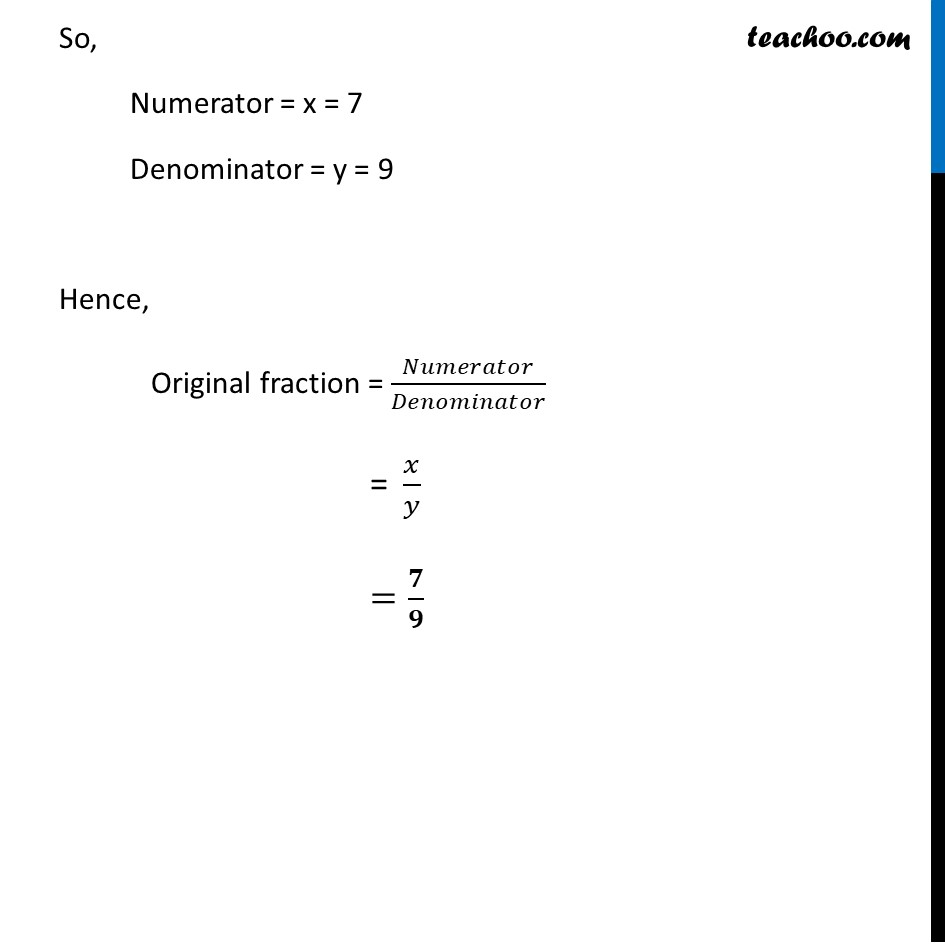Learn in your speed, with individual attention - Teachoo Maths 1-on-1 Class

### Transcript

Ex 3.2, 3 Form the pair of linear equations for the following problems and find their solution by substitution method. (v) A fraction becomes 9/11 , if 2 is added to both the numerator and the denominator. If, 3 is added to both the numerator and the denominator it becomes 5/6 . Find the fraction. Let Numerator be x & Denominator be y So, fraction is 𝒙/𝒚 Given that If 2 is added to both the numerator and the denominator, fraction becomes 9/11 (𝑵𝒖𝒎𝒆𝒓𝒂𝒕𝒐𝒓 + 𝟐)/(𝑫𝒆𝒏𝒐𝒎𝒊𝒏𝒂𝒕𝒐𝒓 + 𝟐)=𝟗/𝟏𝟏 (𝑥 + 2)/(𝑦 + 2)=9/11 11(x + 2) = 9(y + 2) 11x + 22 = 9y + 18 11x – 9y = 18 − 22 11x – 9y = −4 Also, Given that if 3 is added to both the numerator and the denominator, fraction becomes 5/6 (𝑵𝒖𝒎𝒆𝒓𝒂𝒕𝒐𝒓 + 𝟑)/(𝑫𝒆𝒏𝒐𝒎𝒊𝒏𝒂𝒕𝒐𝒓 + 𝟑)=𝟓/𝟔 (𝑥 + 3)/(𝑦 + 3)=5/6 6(x + 3) = 5(y + 3) 6x + 18 = 5y + 15 6x – 5y = 15 − 18 6x – 5y = −3 Hence, our equations are 11x – 9y = −4 …(1) 6x – 5y = −3 …(2) From (1) 11x – 9y = −4 11x = 9y – 4 x = ((𝟗𝒚 − 𝟒)/𝟏𝟏) Putting x in (2) 6x – 5y + 3 = 0 6 ((9𝑦 − 4)/11) – 5y + 3 = 0 Multiply both sides by 11 11 ×(6 (9𝑦 − 4))/11 −5𝑦×11+3×11=0×11 6(9y – 4 ) – 55y + 33 = 0 6(9y) – 6(4) – 55y + 33 = 0 54y – 24 – 55y + 33 = 0 –y + 9 = 0 y = 9 Putting y = 9 in (1) 11x – 9y = −4 11x – 9(9) = −4 11x – 81 = −4 11x = −4 + 81 11x = 77 x = 77/11 x = 7 Therefore x = 7, y = 9 So, Numerator = x = 7 Denominator = y = 9 Hence, Original fraction = 𝑁𝑢𝑚𝑒𝑟𝑎𝑡𝑜𝑟/𝐷𝑒𝑛𝑜𝑚𝑖𝑛𝑎𝑡𝑜𝑟 "= " 𝑥/𝑦 =𝟕/𝟗